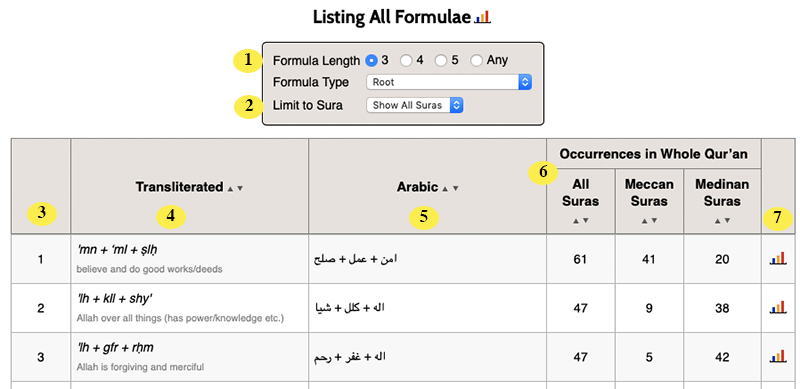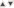# List All Formulae

If you choose  List All Formulae report, found under the “Formulae” menu, Qur’an Gateway will display a list of all the formulaic phrases found in the Arabic text of the Qur’an. (Learn more about formulaic analysis, the terminology used, and some of its implications for the study of the Qur’an here). The List All Formulae report looks like this:1 Formula Length and Type. You can choose the type of formulae that Qur’an Gateway counts and shows in the report. (The length is the number of Arabic words in a formula; the type allows you to choose from any of the three formula types that Qur’an Gateway understands). Learn more about formula lengths and types here. 2 Limit to Sura. If you wish, you can choose to limit the list of formulae to those found in just one particular sura; to do so, just pick the sura number you are interested in from this pick list. 3 Formulae Count. Helpfully lets you see the row number for each formula in the table. 4 Transliterated Form of the Formula. Shows the formula transliterated into Roman (Latin) letters — the "+" means followed by, so in the first row above, the formula is the root 'mn followed by the root ‘ml followed by the root ṣlḥ. Underneath each transliteration is shown a brief gloss of the formula, or the words in it. (You can turn these formulaic glosses off in Preferences if you would prefer not to see them). 5 Arabic Form of the Formula. Shows the formula in its Arabic form. 6 Occurrences. These three columns show how often each formula occurs in the whole Qur’an (whether in all suras, Meccan suras, or Medinan suras). You can sort the table by any of these columns, should you wish, simply by using the using thebuttons at the top of each of them. 7 Chart. See a chart of how a formula is distributed across the Qur’an by clicking its associatedicon. (Or just point your mouse at theicon for a few seconds to see a “mini chart” giving you a quick look at the information).